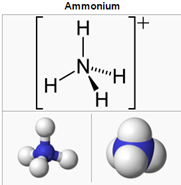﻿

# How can I convert between NH4 (ammonium) to NH3 (ammonia)?

## Document ID

Document ID TE8669

## Published Date

Published Date 07/15/2020
Question
How can I convert between NH4 (ammonium) to NH3 (ammonia)?
Summary
NH4-N to NH4 and NH4 to NH3 conversions.
Ammonium has a chemical formula consisting of (1) Nitrogen and (4) Hydrogen molecules:
NH4The molecular weight of NH4  is calculated as below:
N = 14.0067
H = 1.0079
So, the molecular weight of NH4  would be 14.0067 + (1.0079 X 4) = 18.0383

• To convert the measured value of NH4 –N to NH4, use the following conversion formula:
NH4– N X 1.2878 (derived by dividing the molecular weight of NH4  by N) = NH4.

• Using the equation below, you can get a pretty close approximation for converting NH4 to NH3:
NH4 X 0.9441 (derived by dividing the molecular weight of NH3  by NH4) = approximate NHconcentration.